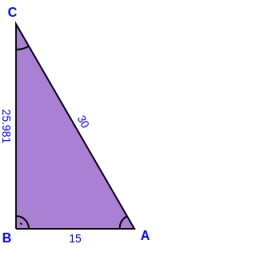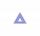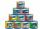# Land boundary

The land has the shape of a right triangle. The hypotenuse has a length of 30m. The circumference of the land is 72 meters. What is the length of the remaining sides of the land boundary?

Result

a =  24 m
b =  18 m

#### Solution:

$c = 30 \ m \ \\ o = 72 \ m \ \\ \ \\ c^2 = a^2+b^2 \ \\ o = a+b+c \ \\ c^2 = a^2 + (o-a-c)^2 \ \\ \ \\ 30^2 = a^2 + (42-a)^2 \ \\ \ \\ \ \\ -2a^2 +84a -864 = 0 \ \\ 2a^2 -84a +864 = 0 \ \\ \ \\ p = 2; q = -84; r = 864 \ \\ D = q^2 - 4pr = 84^2 - 4\cdot 2 \cdot 864 = 144 \ \\ D>0 \ \\ \ \\ a_{1,2} = \dfrac{ -q \pm \sqrt{ D } }{ 2p } = \dfrac{ 84 \pm \sqrt{ 144 } }{ 4 } \ \\ a_{1,2} = \dfrac{ 84 \pm 12 }{ 4 } \ \\ a_{1,2} = 21 \pm 3 \ \\ a_{1} = 24 \ \\ a_{2} = 18 \ \\ \ \\ \text{ Factored form of the equation: } \ \\ 2 (a -24) (a -18) = 0 \ \\ a = a_{ 1 } = 24 = 24 \ \text { m }$

Checkout calculation with our calculator of quadratic equations.

$b = a_{ 2 } \ \\ \ \\ b = o-a-c = 72-24-30 = 18 = 18 \ \text { m }$

Try calculation via our triangle calculator.Our examples were largely sent or created by pupils and students themselves. Therefore, we would be pleased if you could send us any errors you found, spelling mistakes, or rephasing the example. Thank you!

Leave us a comment of this math problem and its solution (i.e. if it is still somewhat unclear...):Be the first to comment!#### Following knowledge from mathematics are needed to solve this word math problem:

Do you have a linear equation or system of equations and looking for its solution? Or do you have quadratic equation? Pythagorean theorem is the base for the right triangle calculator. Do you want to convert length units? See also our trigonometric triangle calculator.

## Next similar math problems:

1. Spruce heightHow tall was spruce that was cut at an altitude of 8m above the ground and the top landed at a distance of 15m from the heel of the tree?
2. Right 24Right isosceles triangle has an altitude x drawn from the right angle to the hypotenuse dividing it into 2 unequal segments. The length of one segment is 5 cm. What is the area of the triangle? Thank you.
3. CathetiThe hypotenuse of a right triangle is 41 and the sum of legs is 49. Calculate the length of its legs.
4. Triangle - is RT?Triangle has a circumference of 90 cm. Side b is 1 cm longer than c, side c is 31 cm longer than side a. Calculate the length of sides and determine whether triangle is a right triangle.
5. Length ITFind the length (circumference) of an isosceles trapezoid in which the length of the bases a,c and the height h are given: a = 8 cm c = 2 cm h = 4 cm
6. Triangle IRTIn isosceles right triangle ABC with right angle at vertex C is coordinates: A (-1, 2); C (-5, -2) Calculate the length of segment AB.
7. Euclid 5Calculate the length of remain sides of a right triangle ABC if a = 7 cm and height vc = 5 cm.
8. Medians and sidesDetermine the size of a triangle KLM and the size of the medians in the triangle. K=(-5; -6), L=(7; -2), M=(5; 6).
9. Triangle ABCIn a triangle ABC with the side BC of length 2 cm The middle point of AB. Points L and M split AC side into three equal lines. KLM is isosceles triangle with a right angle at the point K. Determine the lengths of the sides AB, AC triangle ABC.
10. Cable carCable car rises at an angle 45° and connects the upper and lower station with an altitude difference of 744 m. How long is "endless" tow rope?
11. CalculateCalculate the length of a side of the equilateral triangle with an area of 50cm2.
12. Is right triangleDecide if the triangle XYZ is rectangular: x = 4 m, y = 6 m, z = 4 m
13. Right trianglesHow many right triangles we can construct from line segments 3,4,5,6,8,10,12,13,15,17 cm long? (Do not forget to the triangle inequality).The double ladder shoulders should be 3 meters long. What height will the upper top of the ladder reach if the lower ends are 1.8 meters apart?Side b is 2 cm longer than side c, side a is 9 cm shorter than side b. The triangle circumference is 40 cm. Find the length of sides a, b, c . .. .We want to prove the sentence: If the natural number n is divisible by six, then n is divisible by three. From what assumption we started?How many cans must be put in the bottom row if we want 182 cans arrange in 13 rows above so that each subsequent row has always been one tin less? How many cans will be in the top row?